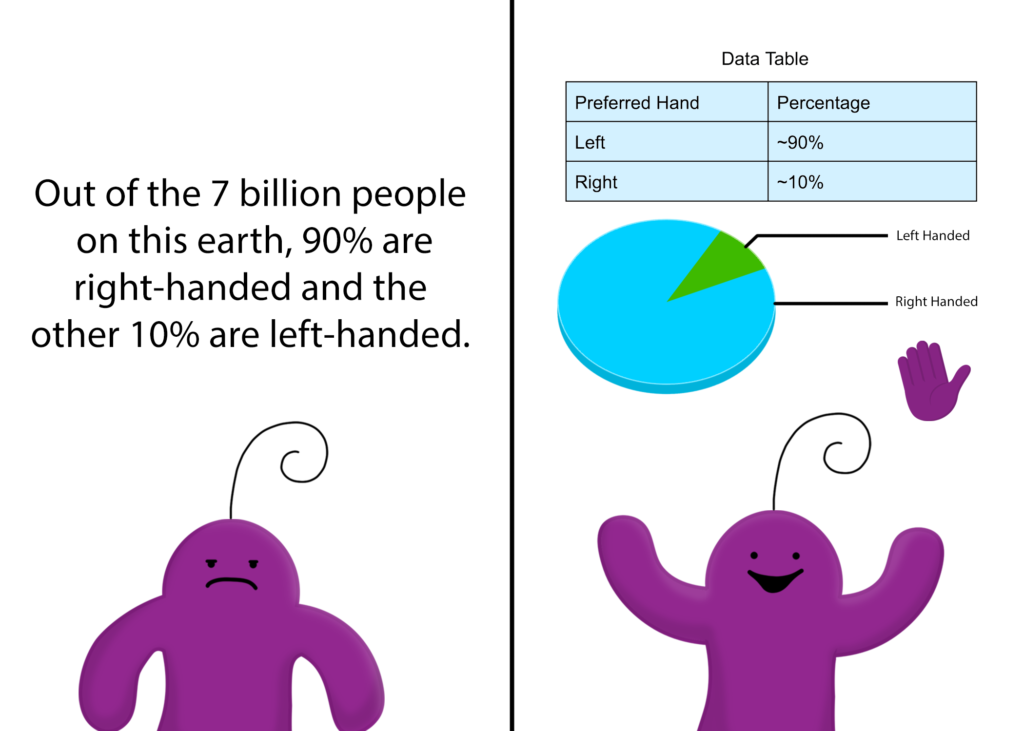# How to Tell a Story Using… Numbers (Part 2)

Last time, we talked about using numbers in our storytelling. If you haven’t read that post yet, check that out here! This week, we’ll be going over part 2 of the same topic. Keep an eye out for future installments in this series!

In my last blog post, I went over 5 of my favorite ways to incorporate numbers into compelling storytelling. And while I did give you resources to create your own graphs and charts, I didn’t quite explain that there are many different types of graphs and charts you can make! Each of these types display data differently and are used for different things. That’s why it is so important to choose the right graph to display your data!

Today, I’ll be going over 10 different basic graphs and charts. Next week we will show you examples of each one.

1. Bar chart – These are plotted vertically or horizontally (usually vertically). Bars of different heights and colors are arranged in a straight line. The heights match up with a numbered line to show what is being measured. This type of graph is usually used to compare values across different groups in your data.
2. Stacked bar chart – Similar to bar charts, stacked bar charts involve bars of different heights and colors arranged in a straight line. However, each bar on the graph is subdivided into several variables of differing heights. .
3. Histogram – Commonly confused with a bar chart, histograms are (yet again!) made with a series of bars on a straight line. However, histograms are used to show the change in values of a single group  over increments of time.
4. Line graph – Likely most synonymous with the word “trends”, a line graph is simply a line plotted onto a coordinate graph. The line can be formed by plotting based on the y- and x- values of each data point.  Line graphs can be used for visualizing trends over time as long as you have two variables.
5. Scatter plot – Scatter plots are very similar to line graphs, but on these types of charts,  the individual points representing data are not connected with a line. Scatter plots are better than line graphs for showing the distribution of data based on two variables, where clusters of data points can be spotted quickly and easily.
6. Proportional Area chart – A proportional area chart is made up of several squares, each of which represents a different figure. Each square’s area is proportional to the value it represents. This type of chart is amazing for visualizing the sizes or amounts of different things.
7. Bubble chart – A bubble chart is a mix between a scatter plot and a proportional area chart. Proportional area charts show the relative sizes of different groups, while scatter plots show the distribution of data. A bubble chart can therefore show the relationship between 3 different variables.
8. Pie chart – This type of graph is a big circle divided into different “slices” representing different groups. Pie charts can be used to show a part-to-whole relationship or to compare several groups to each other.
9. Pictogram Graphs – These graphs are easy to make and interpret because they simply  use icons to represent data. They are also known as “pictographs” or “icon charts”.
10. Box plot – A box plot, also called a “whisker plot”, shows spread of numerical data using 5 numbers as a summary. These 5 numbers are the minimum, first quartile, median, third quartile, and maximum. The minimum and maximum are simply the smallest and largest numbers in the data set; the median is the middle number of the data; the first quartile is the middle number between the minimum and median; the third quartile is the middle number between the median and the maximum.

Next time, we’ll be posting some examples of each type of chart or graph. See you then!

Update from the author: In this blog, we went over 10 types of graphs and charts. If you’d like to see some examples of each type, check out this post: https://boldspeaker.com/how-to-tell-a-story-using-numbers-picture-examples/To continue reading this article or receive future blog articles when they are posted, please subscribe.  Please note that we take your privacy seriously and do not share your details with anyone! If you are interested in our ebook, please check out "Public Speaking for Kids - An Illustrated Guide!"